# Train Maths

## Background

• A game played in Sydney trains by bored schoolkids.
• Every train carriage has a four-digit number.
• Use the digits to make ten using plus, minus, multiply and divide.

## Extra Rules

• Using the numbers in order scores higher.
• Brackets are involved
• Some people use other math operations like exponents, roots etc.

## Examples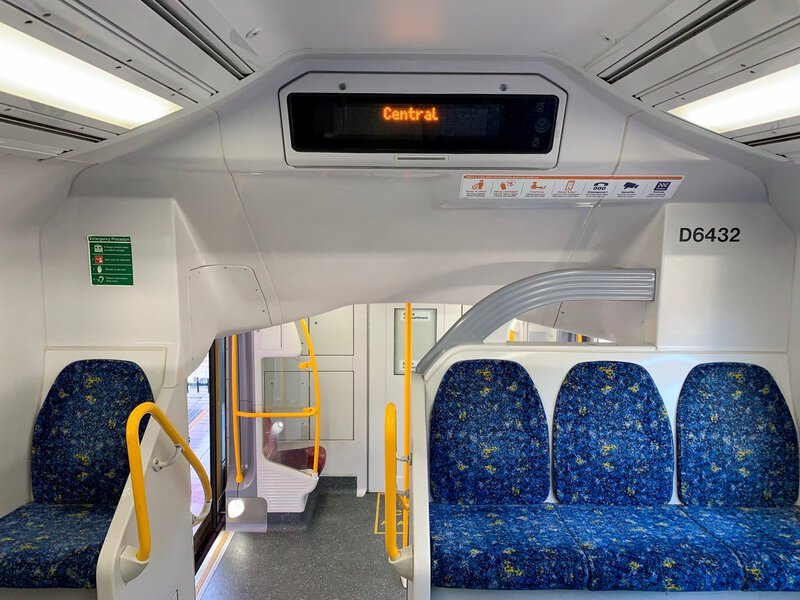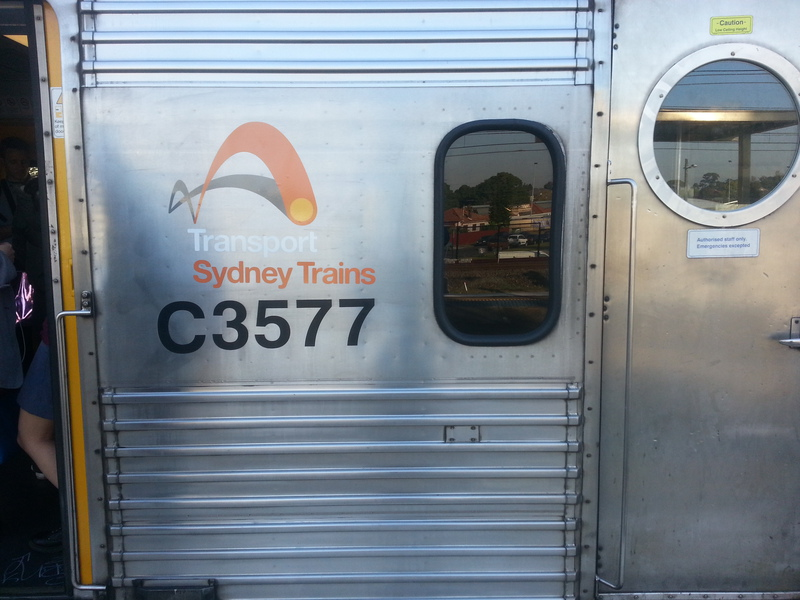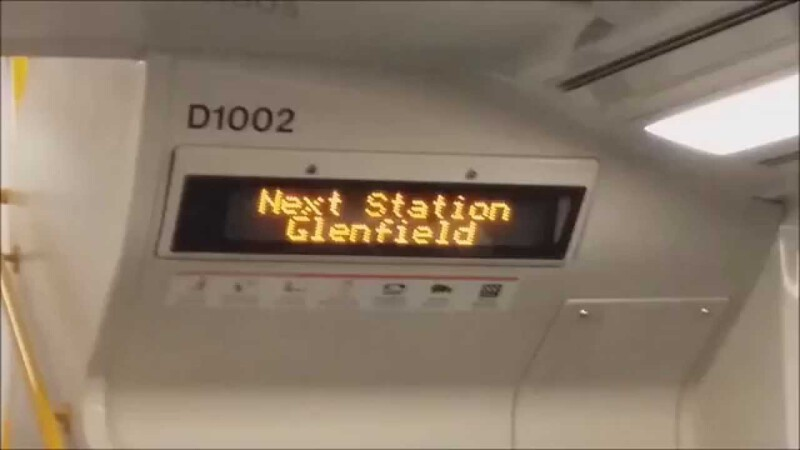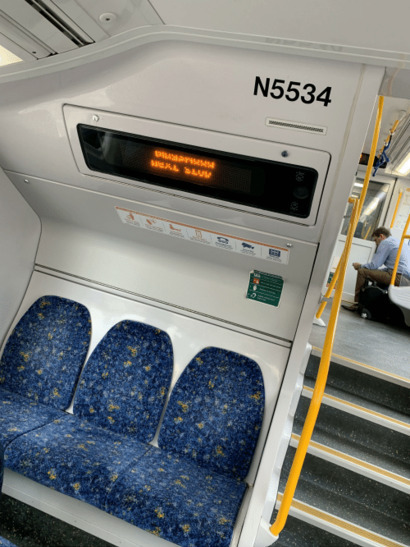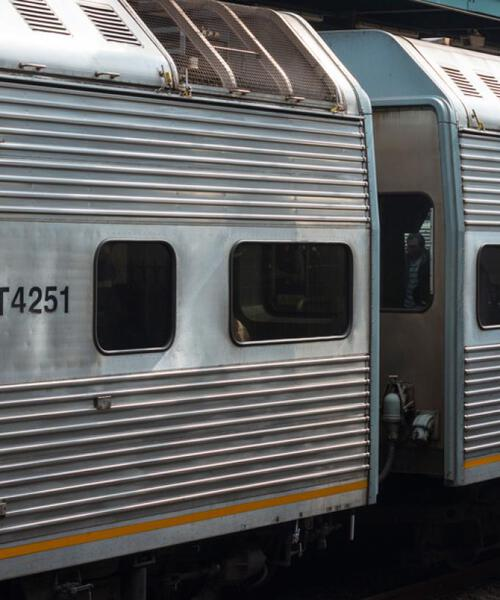### In-order search:

• given four digits
• run through a sequence of calculations with those digits
• stop when you get to one which calculates to 10

### Any-order-search:

• given four digits
• for each permutation of those digits
• run through a sequence of calculations with those digits
• stop when you get to one which calculates to 10

So, lots of calculations…

Some permutations are identical so you can optimise those away

If any of the digits are repeated, some permutations will be identical

1234 has 24 permutations

1231 also has 24 permutations, but some of them are identical, so there are really only 12

It doesn't

## I used Perl modules to pre-calculate all the answers:

Because permutations and combinations are different things.

This script runs it through 768 different eval() calls: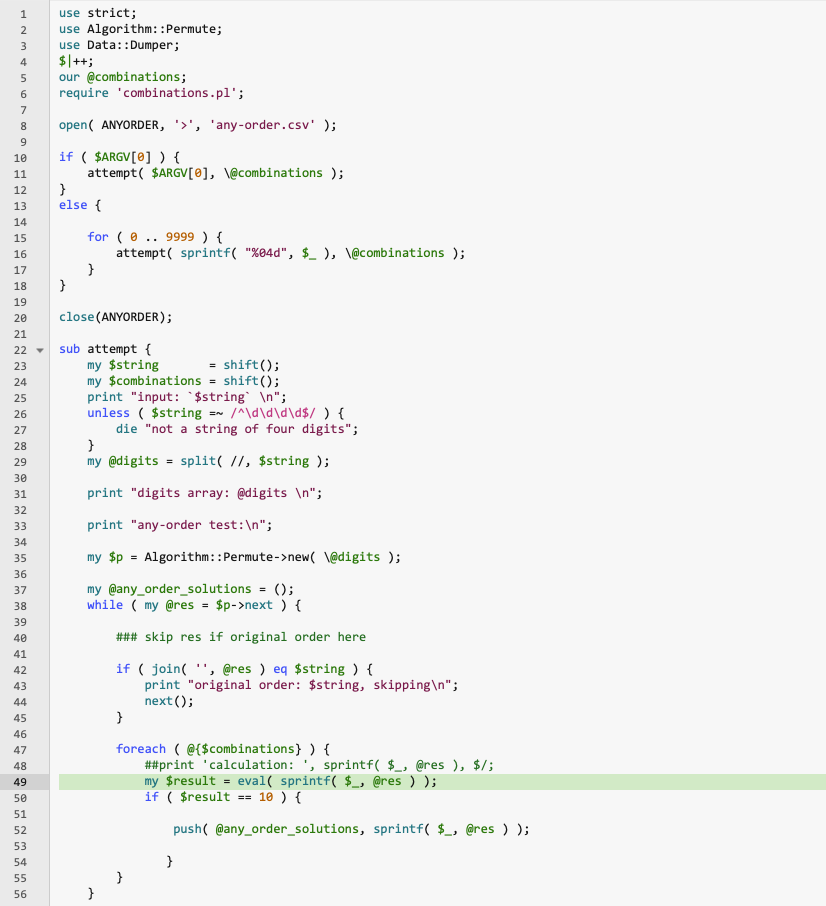The strings for those eval() calls were in turn generated by this script: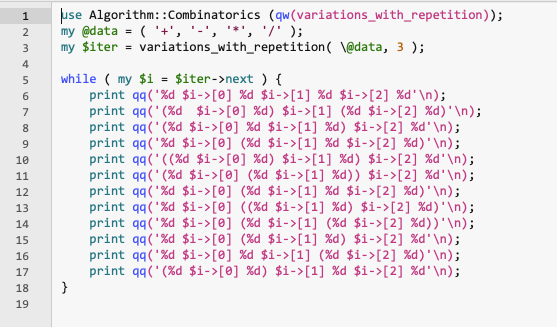To create this library of possible combinations: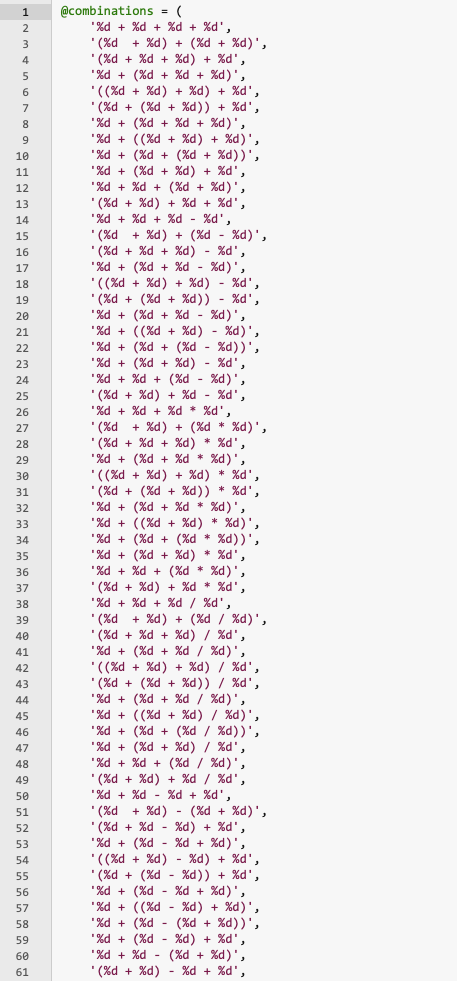## So what is the javascript doing while it 'finds' the answer?

Nothing!

It's just a better user experience if you feel like the website is really working hard finding your answer…

/